# Quantitative Analysis

This section describes methods for the quantitative analysis of objects in binary images, including counting, measuring distances, and analyzing other object properties.

Particle Measurements

The following functions deal with various kinds of particle measurements, such as counting, clamping (measuring distances), and making other more complex measurements.

Counting Objects

The function IMAQ Count Objects is again a complete image analysis application, similar to the function IMAQ Find Horizontal Edge we used in Exercise 5.6. It is possible to adjust various parameters, such as the search rectangle, the type of objects (bright or dark), whether holes in objects should be filled, or whether objects touching the border of the image are to be ignored. Because the function requires a gray-level image as input, the threshold value also has to be specified.

Exercise 5.12: Counting Objects.

From Exercise 5.6 (one of the "locating edges" exercises), replace the IMAQ function by IMAQ Count Objects (you will find the VI in Motion and Vision / Machine Vision / Count and Measure Objects). Add controls for the search rectangle and a cluster control for the settings, similar to the previous exercises. See Figure 5.36 for the panel and Figure 5.37 for the diagram.

The bottom-left corner of Figure 5.36 shows the objects to count in IMAQ Vision Builder. The function IMAQ Count Objects shows the bounding box for each object, the center of each object, and the search area specified by the Search Rectangle cluster control.

Figure 5.36. Counting Objects in Gray-Scaled ImagesFigure 5.37. Diagram of Exercise 5.12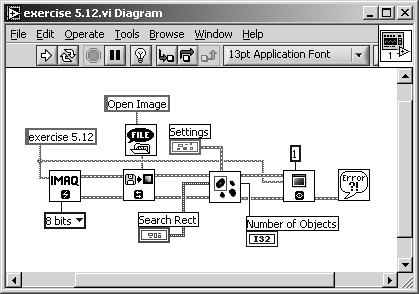Note that not only the big objects, such as the bear's face, are found, but also very small objects, which are hardly visible in the left part of Figure 5.36. The range within objects that should be counted is adjusted by the controls Minimum Object Size and Maximum Object Size.

Measuring Distances (Clamping)

For our next exercise we examine a group of functions located in Motion and Vision / Machine Vision / Measure Distances. They are IMAQ Clamp Horizontal Max , IMAQ Clamp Horizontal Min , IMAQ Clamp Vertical Max , and IMAQ Clamp Vertical Min .

Again, these functions are complete vision applications and provide a number of outputs and image overlays, similar to the "locating edge" examples. Using these VIs, we can measure the extensions of objects in gray-scaled images, either in a horizontal or vertical direction.

Exercise 5.13: Clamping Distances.

Modify Exercise 5.6 (one of the "locating edges" exercises) by replacing the IMAQ function by IMAQ Clamp Vertical Max . Add controls for the search rectangle and a cluster control for the settings, as in the previous exercises.

In a next step, add a case structure to the VI, so that it is possible to select the desired function. Add a Text Ring control so that you can specify the function through the front panel. You can also add a numeric indicator for displaying the measured distance. See Figure 5.38 for panel, diagram, and a first result showing the measuring of vertical maximum distances.

Figure 5.38. Measuring Vertical Maximum Distances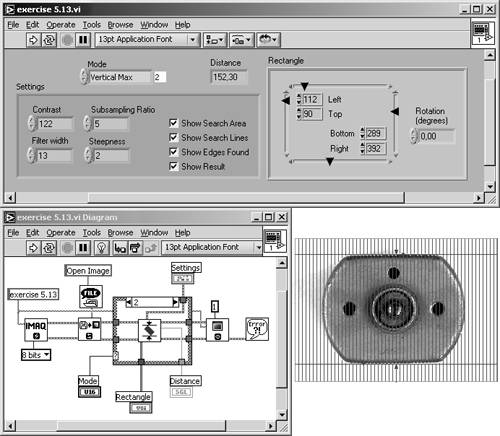Naturally, the term "objects in gray-scaled images" I used a few sentences before is not correct, and you already know why: it is necessary to specify a threshold level to convert the gray-scaled image to a binary one. After that, you will find areas with pixel value 1 and other areas with pixel value 0; it depends on you, which of them you call "objects" and which of them "background."

The clamp functions use parameters called Contrast, Filter Width, Subsampling Ratio, and Steepness to define the contours of the objects that must be measured. See Figures 5.39, 5.40, and 5.41 for measuring vertical minimum distances and horizontal maximum and minimum distances, respectively.

Figure 5.39. Measuring Vertical Minimum Distances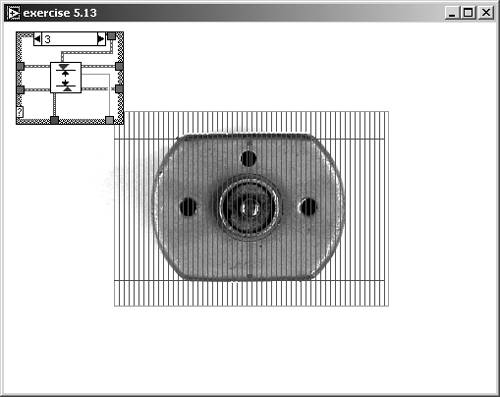Figure 5.40. Measuring Horizontal Maximum Distances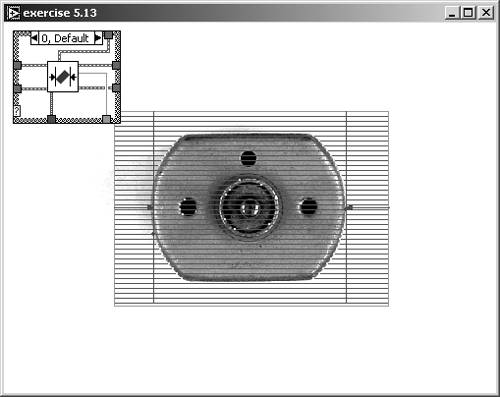Figure 5.41. Measuring Horizontal Minimum Distances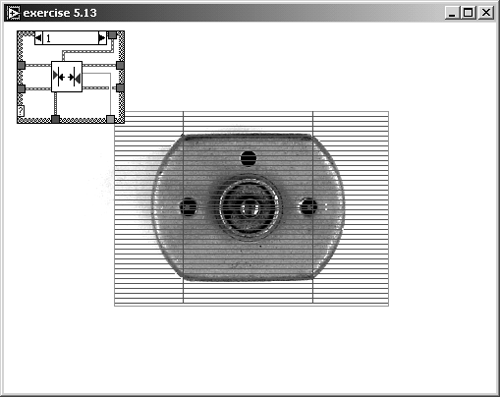Complex Particle Measurements

The next two functions we discuss in this section, IMAQ BasicParticle and IMAQ ComplexParticle , can be used for a detailed analysis of particles (objects) in binary images. The basic particle function provides only the following information:

• Area (pixels): Surface area of particle in pixels;
• Area (calibrated): Surface area of particle in user units;
• Global Rectangle, defined by the limits

• left value,
• top value,
• right value, and
• bottom value.

Exercise 5.14: Basic Particle Analysis.

Start with Exercise 4.16 and add the function IMAQ BasicParticle . Change the Selection Values control to a constant, and filter the image so that not too many particles remain in your image. Add an indicator to display Basic Particle Reports .

Figure 5.42 shows the panel of the exercise, the filtered image, and the labeled particles. I used IMAQ Vision Builder for the labeling, but you could include the labeling in this exercise. See also Figure 5.43 for the diagram window.

Figure 5.42. Basic Particle Analysis of a Filtered Image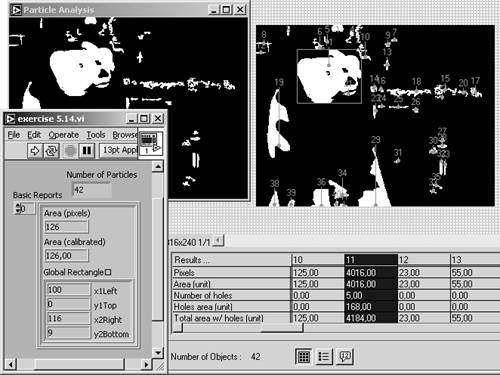Figure 5.43. Diagram of Exercise 5.14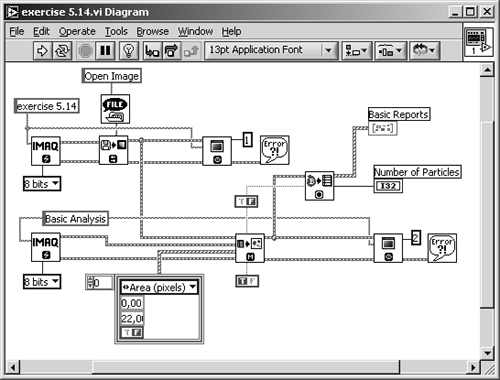For our next exercise, which uses the function IMAQ ComplexParticle , we simply replace the function IMAQ BasicParticle in Exercise 5.14. The Complex Particle function provides the following parameters:

• Area (pixels);
• Area (calibrated);
• Perimeter: Length of outer contour of particle in user units;
• Number of holes;
• Holes area: Surface area of holes in user units;
• Hole perimeter: Perimeter of all holes in user units;
• Global rectangle;
• SumX: Sum of the x axis for each pixel of the particle;
• SumY: Sum of the y axis for each pixel of the particle;
• SumXX: Sum of the x axis squared for each pixel of the particle;
• SumYY: Sum of the y axis squared for each pixel of the particle;
• SumXY: Sum of the x axis and y axis for each pixel of the particle;
• Longest segment coordinates with

• Longest segment (X) and
• Longest segment (Y);
• Projection X;
• Projection Y.

Exercise 5.15: Complex Particle Analysis.

Replace the IMAQ Vision function IMAQ BasicParticle in Exercise 5.14 with IMAQ ComplexParticle and modify the indicator, here called Complex Reports, to display the results. See Figure 5.44 and Figure 5.45.

Figure 5.44. Complex Particle Analysis of a Filtered Image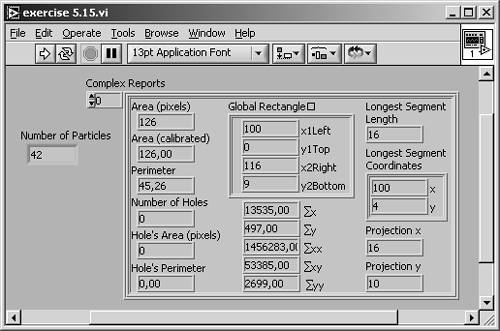Figure 5.45. Diagram of Exercise 5.15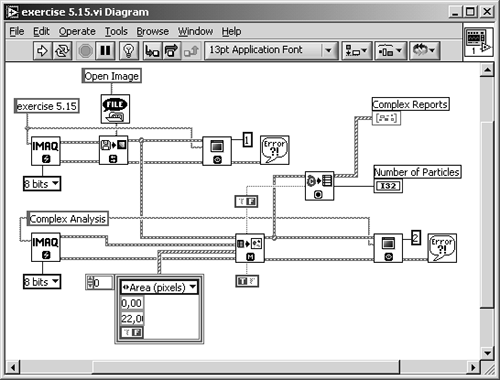IMAQ ChooseMeasurements , another function of this group, filters the reports generated either by IMAQ BasicParticle or by IMAQ ComplexParticle , based on a selection parameter and minimum and maximum values. We need not try this function in an exercise here, because our VIs already provide particle filtering with the function IMAQ ParticleFilter .

To calculate the whole range of particle parameters, or at least one of the more complex ones we mentioned in Chapter 4, you use the function IMAQ ComplexMeasure .

Add the IMAQ Vision function IMAQ ChooseMeasurements to Exercise 5.15. Provide a control for choosing the parameter you want to measure, and provide a numeric 1D-array for the results. See Figure 5.46 and Figure 5.47.

Figure 5.46. Calculating Other Complex Particle Parameters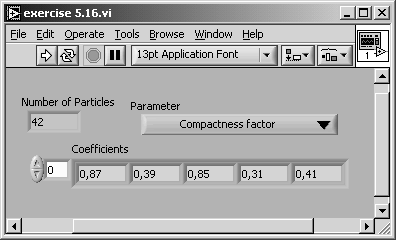Figure 5.47. Diagram of Exercise 5.16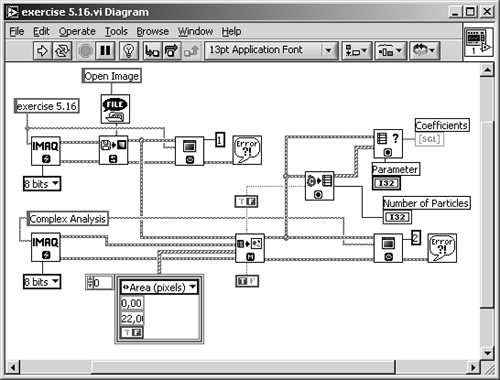In the next pages we discuss in more detail the parameters that can be measured by IMAQ ComplexParticle or calculated by IMAQ ChooseMeasurements . You can also find these details in .

• Area (pixels): Surface area of particle in number of pixels. Holes in particles are not included.
• Area (calibrated) A: Surface area of particle in user units. We learn about calibration of images later in this chapter.
• Number of holes: Number of holes within a particle.
• Holes' area A h : Surface area of all holes within a particle.
• Total area A t : Area of particle including areas of all holes:

Equation 5.1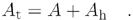• Scanned area A s : Surface area of the entire image in user units:

Equation 5.2• Ratio area/scanned area R p (%): Percentage of the image occupied by particles:

Equation 5.3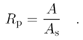• Ratio area/total area R t (%): Percentage of particle surface in relation to the total area:

Equation 5.4• Center of mass (X): x coordinate of the center of gravity. In general, the center of gravity G of a particle, which consists of n pixels P i , is defined by

Equation 5.5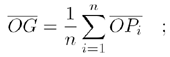the x coordinate of G is therefore

Equation 5.6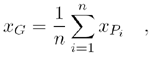which is the average location of the central points of the horizontal segments in a particle .

• Center of mass (Y): y coordinate of the center of gravity:

Equation 5.7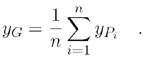• Left column (X): Left x coordinate of bounding rectangle;
• Upper row (Y): Top y coordinate of bounding rectangle;
• Right column (X): Right x coordinate of bounding rectangle;
• Lower row (Y): Bottom y coordinate of bounding rectangle. If x i and y i are the coordinates of a pixel P i in a particle, there exists a horizontal rectangle defined by two points (min x i , min y i ) and (max x i , max y i ):

Equation 5.8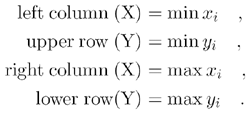• Width l: Width of bounding rectangle:

Equation 5.9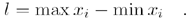• Height h: Height of bounding rectangle:

Equation 5.10• Longest segment length: Length of longest horizontal line segment.
• Longest segment left column (X): Leftmost x coordinate of longest horizontal line segment.
• Longest segment row (Y): y coordinate of longest horizontal line segment.
• Perimeter p: Length of the outer contour of a particle.
• Hole perimeter: Perimeter of all holes in user units.
• SumX: Sum of the x coordinates of each pixel in a particle.
• SumY: Sum of the y coordinates of each pixel in a particle.
• SumXX: Sum of the x coordinates squared for each pixel in a particle.
• SumYY: Sum of the y coordinates squared for each pixel in a particle.
• SumXY: Sum of the x and y coordinates for each pixel in a particle.
• Corrected projection x : Sum of the horizontal segments that do not superimpose any other horizontal segment.
• Corrected projection y : Sum of the vertical segments that do not superimpose any other vertical segment.
• Moment of inertia I xx : All moments of inertia are a representation of the pixel distribution of a particle in relation to its center of gravity. If A is the particle area and x G the x coordinate of the center of gravity, the moment of inertia I xx is defined as

Equation 5.11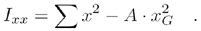• Moment of inertia I yy : If y G is the y coordinate of the center of gravity, the moment of inertia I yy is defined as

Equation 5.12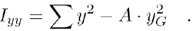• Moment of inertia I xy :

Equation 5.13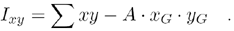• Mean chord X C x : Mean length of horizontal segments in a particle.
• Mean chord Y C y : Mean length of vertical segments in a particle.
• Max intercept S max : Length of longest segment in a particle. All possible directions have to be considered .
• Mean intercept perpendicular C: Mean length of the chords in an object perpendicular to its max intercept. Another description possibility is

Equation 5.14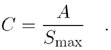• Particle orientation: Direction of the longest segment in a particle. Figure 5.48 shows an example of the last five parameters.

Figure 5.48. Intercept and Chord Particle Measurements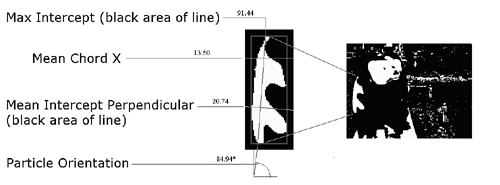• Equivalent ellipse minor axis: Imagine an ellipse having the same area as the particle and a major axis a equal to half the max intercept S max . The minor axis b of this ellipse is

Equation 5.15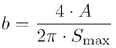if the particle area A is

Equation 5.16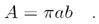• Ellipse major axis: Now imagine another ellipse having the same area and the same perimeter as the particle. The ellipse major axis is the total length of the major axis of this ellipse.
• Ellipse minor axis: Total length of minor axis having the same area and perimeter as the particle.
• Ratio of equivalent ellipse axis R e : Fraction of major axis to minor axis of the equivalent ellipse:

Equation 5.17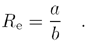• Rectangle big side: Length of the large side of a rectangle having the same area and perimeter as the particle.
• Rectangle small side: Length of the small side of a rectangle having the same area and perimeter as the particle.
• Ratio of equivalent rectangle sides: Ratio of rectangle big side to rectangle small side.
• Elongation factor F e : The ratio of the longest segment in a particle to the mean length of the perpendicular segments:

Equation 5.18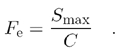• Compactness factor F c : The ratio of the particle area A to the area of the bounding rectangle (always between 0 and 1; or equal to 1 if the particle is rectangular):

Equation 5.19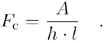• Heywood circularity factor F H : The ratio of the particle perimeter p to the perimeter of a circle having the same area A as the particle:

Equation 5.20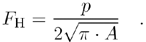• Type factor F t : A complex factor relating the surface area A to the moment of inertia:

Equation 5.21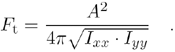• Hydraulic radius R h : The ratio of the particle area A to the particle perimeter p :

Equation 5.22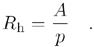• Waddel disk diameter R d : Diameter of the disk having the same area as the particle:

Equation 5.23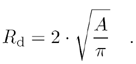• Diagonal d: Diagonal of the bounding rectangle:

Equation 5.24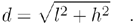Image Calibration

Sometimes it is necessary to convert the x and y dimensions of an image into real units; for example, in our motor image, we can measure some "real" distances on the motor itself.

Before we start a new exercise, let us look at the relevant functions in IMAQ Vision Builder. Load a binary motor image ( dc_motor_bin3.png ) and select Image / Simple Calibration. Use the menu now displayed to define two main topics:

• Origin and orientation: You can draw a line in the image and thus define the origin and the x -axis orientation of a new coordinate system. If the object to measure is already in a correct orientation and you want to measure only distances, not absolute coordinates (which is true for our motor image), you can skip this menu item.
• Pixel calibration: Here, you can define a factor for the x and the y direction as well as the unit for the new dimensions (in our example, "millimeter" is appropriate). You can also set different scaling factors for x and y direction (see Figure 5.49).

Figure 5.49. Calibrating the Motor Image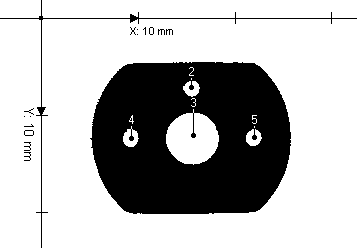With the motor image selected, draw a vertical line from the top to the bottom of the motor, thus specifying the smallest possible distance; this action returns an (image) distance of about n = 150 pixels. The real distance is about d = 15.4 mm, which you must enter in the appropriate control.

The new dimension is now displayed in the coordinate system. The image is now ready for simple or complex particle analysis, which is based on user units; for example, measuring height, width, area, perimeter, and some other parameters in real-world units.

Exercise 5.17: Image Calibration.

Here, we try image calibration in a LabVIEW exercise: In Exercise 5.16, insert the function IMAQ Set Simple Calibration (you can find it in Motion and Vision / Vision Utilities / Calibration) between IMAQ Particle Filter (which is not really necessary here) and IMAQ WindDraw . Provide an additional control for setting the grid description. As mentioned above, it is not necessary here to change orientation and origin of the coordinate system. See Figure 5.50 and Figure 5.51.

Figure 5.50. Setting a Simple Pixel CalibrationFigure 5.51. Diagram of Exercise 5.17We can calculate the correct factors "X Step" and "Y Step" in Exercise 5.17 by using the values we obtained from IMAQ Vision builder. The value f for both directions is

Equation 5.25Another possibility is to use a grid image , showing circular dots specifying the distortion of an image (Figure 5.52). Calibration of the image (we use IMAQ Vision Builder here) is done by the following steps:

Figure 5.52. Grid Calibration with IMAQ Vision Builder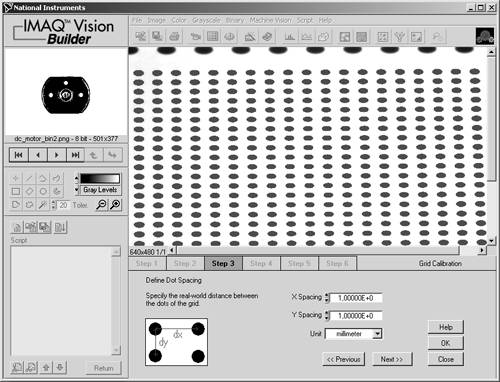1. The template image, showing the calibration grid, is loaded.
2. The grid image is converted into a binary image. An appropriate threshold must be specified.
3. The real-world distance between the grid dots in x and y direction must be specified.
4. A new coordinate system can be defined, similar to the system in simple calibration example (Figure 5.49).
5. In case of a perspective or nonlinear distortion, these parameters must be specified.
6. The calibration data is now learned and can be used to display corrected images.

### Shape Matching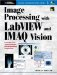Image Processing with LabVIEW and IMAQ Vision
ISBN: 0130474150
EAN: 2147483647
Year: 2005
Pages: 55